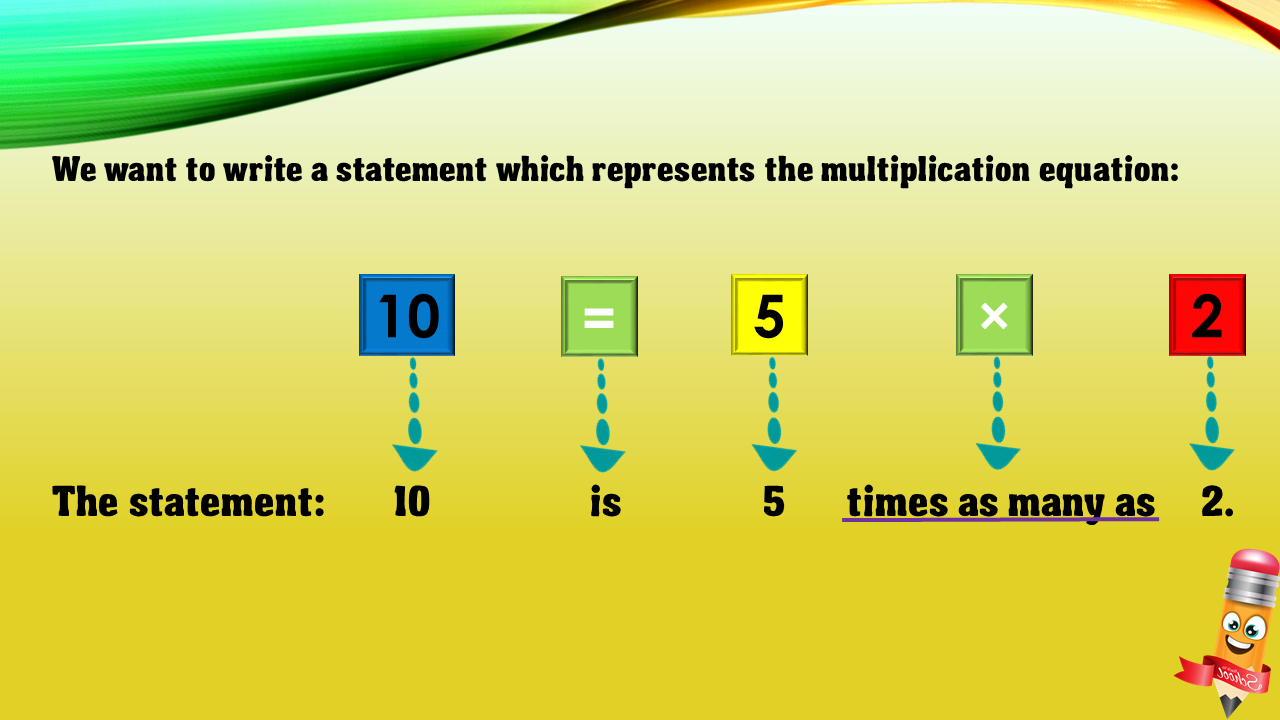1
visibility

Choose one statement which represents the equation: 10 = 5×2?

• A

10 times as many as 5 is 2.

• B

10 is 5 more than 2.

• C

10 is 5 times as many as 2.

• D

5 is 2 less than 10.